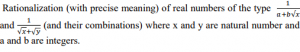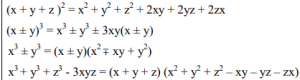# Class IX Maths Practice – Notes, Sample Papers & Worksheets

CLASS 9 MARKING SCHEME ( CBSE 2020 )

 Units Unit Name Marks I NUMBER SYSTEMS 8 II ALGEBRA 17 III COORDINATE GEOMETRY 4 IV GEOMETRY 28 V MENSURATION 13 VI STATISTICS & PROBABILITY 10 Total 80 Internal assessment I20 Grand Total 100

CHAPTER 1 : REAL NUMBERS

Review of representation of natural numbers, integers and rational numbers on the number line. Representation of terminating / non terminating recurring decimals on the number line through successive magnification. Rational numbers as recurring/ terminating decimals. Operations on real numbers. Examples of non-recurring/non-terminating decimals. Existence of non-rational numbers (irrational numbers) such as √2, √3 and their representation on the number line. Explaining that every real number is represented by a unique point on the number line and conversely, viz. every point on the number line represents a unique real number. Existence of √x for a given positive real number x and its representation on the number line with geometric proof. Definition of nth root of a real number.Recall of laws of exponents with integral powers. Rational exponents with positive real bases (to be done by particular cases, allowing learner to arrive at the general laws.)

Class 9 Maths Key Notes with Question Answers ( Unit – Number System )

Roots of Real Numbers Worksheet – Class 9 Maths

CHAPTER 2 : POLYNOMIALS

Definition of a polynomial in one variable with examples and counter examples. Coefficients of a polynomial, terms of a polynomial and zeroes of polynomial. Degree of a polynomial. Constant, linear, quadratic and cubic polynomials. Monomials, binomials, trinomials. Factors and multiples. Zeros of a polynomial. Motivate and State the Remainder Theorem with examples. Statement and proof of the Factor Theorem. Factorization of ax2 + bx + c, a ≠ 0 where a, b and c are real numbers, and of cubic polynomials using the Factor Theorem. Recall of algebraic expressions and identities. Verification of identities:and their use in factorization of polynomials.

Class 9 Maths Key Notes With Question Answers ( Chapter – Polynomials )

Polynomials Worksheet – Class 9 Maths

Algebraic Identities Worksheet – Class 9 Maths

CHAPTER 3: COORDINATE GEOMETRY

The Cartesian plane, coordinates of a point, names and terms associated with the coordinate plane, notations, plotting points in the plane.

Class 9 Maths Key Notes With Question Answers ( Chapter – Coordinate Geometry )

CHAPTER 4: LINEAR EQUATIONS IN TWO VARIABLES

Recall of linear equations in one variable. Introduction to the equation in two variables. Focus on linear equations of the type ax+by+c=0. Prove that a linear equation in two variables has infinitely many solutions and justify their being written as ordered pairs of real numbers, plotting them and showing that they lie on a line. Graph of linear equations in two variables. Examples, problems from real life, including problems on Ratio and Proportion and with algebraic and graphical solutions being done simultaneously.

Class 9 Maths Key Notes With Question Answers ( Chapter – Linear Equation in two variables )

Linear Equations Worksheet – Class 9 Maths

CHAPTER 5: INTRODUCTION TO EUCLID’S GEOMETRY

History – Geometry in India and Euclid’s geometry. Euclid’s method of formalizing observed phenomenon into rigorous Mathematics with definitions, common/obvious notions, axioms/postulates and theorems. The five postulates of Euclid. Equivalent versions of the fifth postulate. Showing the relationship between axiom and theorem, for example: (Axiom) 1. Given two distinct points, there exists one and only one line through them. (Theorem) 2. (Prove) Two distinct lines cannot have more than one point in common.

Class 9 Maths Key Notes With Question Answers ( Chapter – Introduction to Euclid’s Geometry )

CHAPTER 6: LINES AND ANGLES

1. (Motivate) If a ray stands on a line, then the sum of the two adjacent angles so formed is 180 Degree and the converse.

2. (Prove) If two lines intersect, vertically opposite angles are equal.

3. (Motivate) Results on corresponding angles, alternate angles, interior angles when a transversal intersects two parallel lines.

4. (Motivate) Lines which are parallel to a given line are parallel.

5. (Prove) The sum of the angles of a triangle is 180 Degree .

6. (Motivate) If a side of a triangle is produced, the exterior angle so formed is equal to the sum of the two interior opposite angles.

Class 9 Maths Key Notes With Question Answers ( Chapter – Lines & Angles )

CHAPTER 7:TRIANGLES

1. (Motivate) Two triangles are congruent if any two sides and the included angle of one triangle is equal to any two sides and the included angle of the other triangle (SAS Congruence).

2. (Prove) Two triangles are congruent if any two angles and the included side of one triangle is equal to any two angles and the included side of the other triangle (ASA Congruence).

3. (Motivate) Two triangles are congruent if the three sides of one triangle are equal to three sides of the other triangle (SSS Congruence).

4. (Motivate) Two right triangles are congruent if the hypotenuse and a side of one triangle are equal (respectively) to the hypotenuse and a side of the other triangle. (RHS Congruence)

5. (Prove) The angles opposite to equal sides of a triangle are equal.

6. (Motivate) The sides opposite to equal angles of a triangle are equal.

7. (Motivate) Triangle inequalities and relation between ‘angle and facing side’ inequalities in triangles.

Class 9 Maths Key Notes With Question Answers ( Chapter – Triangles )

1. (Prove) The diagonal divides a parallelogram into two congruent triangles.

2. (Motivate) In a parallelogram opposite sides are equal, and conversely.

3. (Motivate) In a parallelogram opposite angles are equal, and conversely.

4. (Motivate) A quadrilateral is a parallelogram if a pair of its opposite sides is parallel and equal.

5. (Motivate) In a parallelogram, the diagonals bisect each other and conversely.

6. (Motivate) In a triangle, the line segment joining the mid points of any two sides is parallel to the third side and is half of it and  (motivate) its converse.

Class 9 Maths Key Notes With Question Answers ( Chapter – Quadrilaterals )

CHAPTER 9: AREA OF PARALLELOGRAM AND TRIANGLES

Review of concept of Area, Revision of Area of rectangle.

1.(Prove) Parallelograms on the same base and between the same parallels have the same area.

2. (Motivate) Triangles on the same (or equal base) base and between the same parallels are equal in area.

Class 9 Maths Key Notes With Question Answers ( Chapter – Areas of Parallelograms and Triangles )

Class 9 Maths Worksheet – IX ( Areas of Parallelogram and Triangles)

CHAPTER 10: CIRCLES

Through examples, arrive at definition of circle and related concepts radius, circumference, diameter, chord, arc, secant, sector, segment, subtended angle.

1. (Prove) Equal chords of a circle subtend equal angles at the centre and (motivate) its converse.

2. (Motivate) The perpendicular from the centre of a circle to a chord bisects the chord and conversely, the line drawn through the centre of a circle to bisect a chord is perpendicular to the chord.

3. (Motivate) There is one and only one circle passing through three given non-collinear points.

4. (Motivate) Equal chords of a circle (or of congruent circles) are equidistant from the centre (or their respective centres) and conversely.

5. (Prove) The angle subtended by an arc at the centre is double the angle subtended by it at any point on the remaining part of the circle.

6. (Motivate) Angles in the same segment of a circle are equal.

7. (Motivate) If a line segment joining two points subtends equal angle at two other points lying on the same side of the line containing the segment, the four points lie on a circle.

8. (Motivate) The sum of either of the pair of the opposite angles of a cyclic quadrilateral is 180° and its converse.

Class 9 Maths Key Notes With Question Answers ( Chapter Circles )

CHAPTER 11: CONSTRUCTIONS

Construction of bisectors of line segments and angles of measure 60°, 90° , 45° etc., equilateral triangles. Construction of a triangle given its base, sum/difference of the other two sides and one base angle. Construction of a triangle of given perimeter and base angles.

Class 9 Maths Key Notes With Question Answers ( Chapter – Constructions )

CHAPTER 12: HERON’S FORMULA (AREA)

Area of a triangle using Heron’s formula (without proof) and its application in finding the area of a quadrilateral.

Class 9 Maths Key Notes With Question Answers ( Chapter – Heron’s Formula )

CHAPTER 13: SURFACE AREAS AND VOLUMES

Surface areas and volumes of cubes, cuboids, spheres (including hemispheres) and right circular cylinders/cones.

Class 9 Maths Key Notes With Question Answers ( Chapter – Surface Area and Volumes )

Class 9 Maths Worksheet with Answers ( Surface Areas and Volumes )

CHAPTER 14: STATISTICS

Introduction to Statistics: Collection of data, presentation of data — tabular form, ungrouped / grouped, bar graphs, histograms (with varying base lengths), frequency polygons. Mean, median and mode of ungrouped data.

Class 9 Maths Key Notes With Question Answers ( Chapter – Statistics )

CHAPTER 15: PROBABILITY

History, Repeated experiments and observed frequency approach to probability. Focus is on empirical probability. (A large amount of time to be devoted to group and to individual activities to motivate the concept; the experiments to be drawn from  real – life situations, and from examples used in the chapter on statistics)

Class 9 Maths Key Notes With Question Answers ( Chapter – Probability )

Class 9 Mathematics Worksheet – 5 ( Probability )